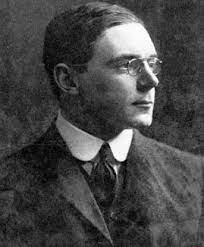A linguist would be shocked to learn that if a set is not closed this does notmean that it is open, or again that “E is dense in E” does not mean thesame thing as “E is dense in itself.”

# Understanding these basic definition and statement~

• We say $A \subset \mathbb{R}$ is open if for every $x \in A$ there exists $\varepsilon>0$ such that $(x-\varepsilon, x+\varepsilon) \subseteq A . A$ is closed if its complement $A^{c}$ is open. Similarly a set $A$ in a metric space $(M, d)$ is called open if for each $x \in A$, there exists an $\varepsilon>0$ such that $B(x ; \varepsilon) \subset A$. Here,
$$B(x ; \varepsilon)={y \in M: d(x, y)<\varepsilon}$$
is the $\varepsilon$-ball (also called $\varepsilon$-neighborhood) around $x$.
• Let $A$ be a subset of a metric space $(M, d)$ and $x \in M$. We say $x$ is an accumulation point
of $A$ if every open set $U$ containing $x$ contains some point $y \in A$ with $y \neq x$.
• Let $A \subset(M, d)$. We say $x \in M$ is a limit point of a set $A$ provided $U \cap A \neq \emptyset$ for every neighborhood $U$ of $x$.
• A set $A$ is closed in a metric space $(M, d)$ if and only if the accumulation points of $A$ belong to $A$ and we set $\bar{A}:=A \cup{x \in M: x$ is an accumulation point of $A}$.

A subset $A$ in a metric space $M$ is called compact if one of the following equivalent conditions is satisfied:

a) Every open cover of $A$ has a finite subcover.
b) Every sequence in $A$ has a convergent subsequence converging to a point in $A$ (sequential compactness).

Furthermore, if $A \in \mathbb{R}^{n}$, the above two conditions are equivalent to saying that $A$ is closed and bounded (Heine-Borel Theorem).

• A metric space $(M, d)$ is called totally bounded if for each $\varepsilon>0$ there is a finite set $\left{x_{1}, x_{2}, \cdot, x_{k}\right}$ in $M$ such that
$$A \subset \bigcup_{i=1}^{k} B\left(x_{i} ; \epsilon\right)$$
• A metric space $(M, d)$ is compact if and only if $M$ is complete and totally bounded.
A subset $A$ of a metric space $(M, d)$ is called nol connecled if there are disjoint open sets $U$ and $V$ such that $A \subseteq U \cup V$ and $A \cap U \neq \emptyset \neq A \cap V .$ Otherwise, the set $A$ is said to be connected.
• A subset $A$ of a metric space $(M, d)$ is said to be path connected if for each pair of points $x$ and $y$ in $A$, there is a path in $A$ connecting $x$ to $y$, i.e., there is a continuous function $\psi:[0,1] \rightarrow A$ such that $\psi(0)=x$ and $\psi(1)=y$
• Let $M$ be a metric space and $A$ be a subset of $M$, and $f: A \rightarrow \mathbb{R}$ be a continuous function. Suppose $B \subset A$ is connected and $x, y \in B$. Then for every real number $c$ such that $f(x)<$ $c<f(y)$, there exists a point $z \in B$ such that $f(z)=c$ Notice that, since intervals (open or closed) are connected, the above statement is a generalized version of the Intermediate Value Theorem given for intervals.

# Try the following problem to test yourself！

Problem 1.

Let $S_{1}=\left[0, \frac{1}{3}\right] \cup\left[\frac{2}{3}, 1\right]$ be obtained from $[0,1]$ by removing the middle third $\left(\frac{1}{3}, \frac{2}{3}\right) .$ Repeat the process to obtain $S_{2}=\left[0, \frac{1}{9}\right] \cup\left[\frac{2}{9}, \frac{1}{3}\right] \cup\left[\frac{2}{3}, \frac{7}{9}\right] \cup\left[\frac{8}{9}, 1\right] .$ In general, $S_{n+1}$ is obtained
from $S_{n}$ by removing the middle third of each interval in $S_{n} .$ Let $C=\bigcap_{n \geq 1} S_{n}$, also known as the Cantor set. Prove that

1. $C$ is compact.
2. $\operatorname{int}(C)=\emptyset$.
3. $C$ has infinitely many points.
4. The total length of the intervals removed is equal to 1 .

Proof .

1. Notice that $C \subset[0,1]$, so it is bounded. $C=\bigcap_{n \geq 1} S_{n}$, where each $S_{n}$ is a union of finitely many closed intervals and so is closed. Thus, the set $C$ is an intersection of closed sets and so $C$ is closed as well. Therefore, $C$ is a closed and bounded subset of $\mathbb{R}$, so $C$ is compact.
2. The length of each of the subintervals making up the set $S_{n}$ is $\frac{1}{3^{n}}$, so the intersection can contain no interval longer than this. Since $\frac{1}{3^{n}} \rightarrow 0$ as $n \rightarrow \infty$, the intersection can contain no interval of positive length. If $a \in C$ is an interior point, we should be able to find an interval around $a$ with positive length $s .$ There are no such intervals, so int $(C)=\emptyset$.
3. Begin by noting that $C$ contains the endpoints of all the intervals for each $S_{n}$ and that each $S_{n}$ has a total of $2^{n+1}$ endpoints. Since the number of endpoints in $S_{n}$ goes to $\infty$ as $n \rightarrow \infty$, we have that $C$ has infinitely many points.
4. By summing up the length of the deleted intervals, we are able to obtain:
$$\frac{1}{3}+\frac{2}{9}+\frac{4}{27}+\cdots=\frac{1}{3}\left[1+\frac{2}{3}+\left(\frac{2}{3}\right)^{2}+\cdots\right]=\frac{1}{3} \cdot \frac{1}{1-\frac{2}{3}}=1$$

Problem 2.

Given a metric space $(X, d)$ and a nonempty bounded subset $A$, the real number $\delta(A):=\sup {d(x, y): x, y \in A}$ iProblem $\mathbf{1 0 . 5 3}$ Let $C$ be a closed subset of $\mathbb{R}$. Show that $C=P \cup F$, where $P$ is perfect, $F$ is countable, and $P \cap F=\emptyset$. This is known as the Cantor-Bendixson Theorem.

Proof .

Let $(X, d)$ be a discrete metric space where $X$ has more than one element. Then
$$S\left(x_{0} ; 1\right)=X \backslash\left{x_{0}\right} \quad \text { and } \quad \delta\left(S\left(x_{0} ; 1\right)\right)=1<2 \text {. }$$ For $r>0$ and $r \neq 1, S\left(x_{0} ; r\right)=\emptyset$ and $\delta\left(S\left(x_{0} ; r\right)\right)$ is not dLet $P$ be the set of all condensed points of $C .$ Set $F=C \backslash P .$ Then $C=P \cup F$. We have $P \cap F=\emptyset$. According to Problem $10.52, P$ is perfect, and $F$ is countable.